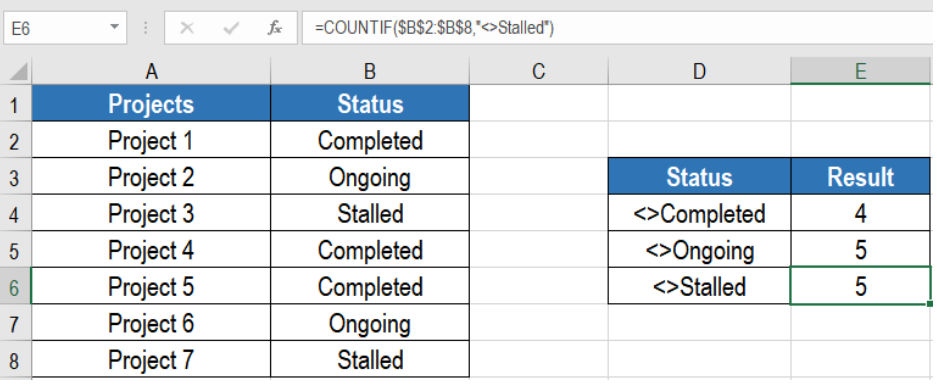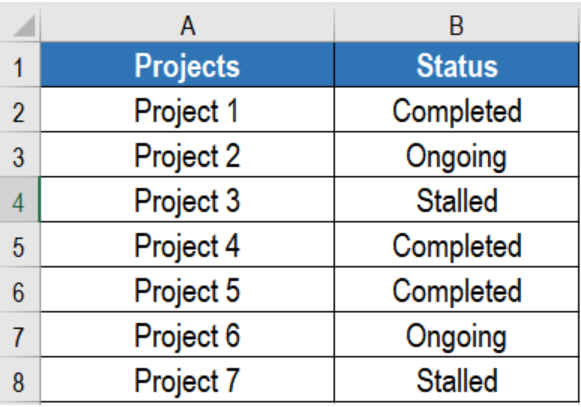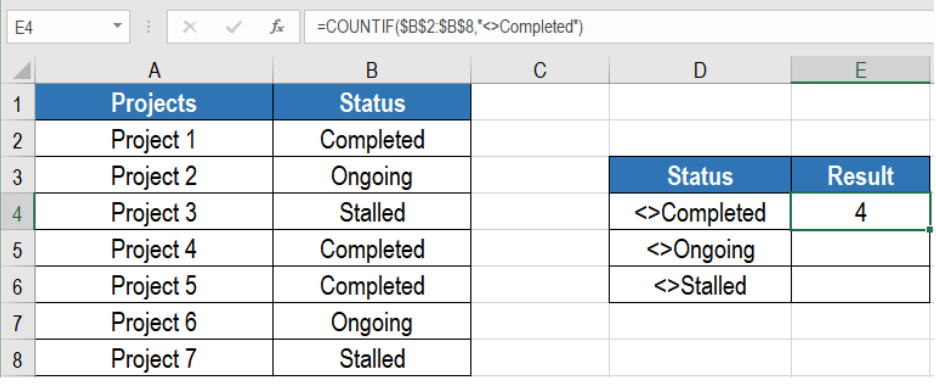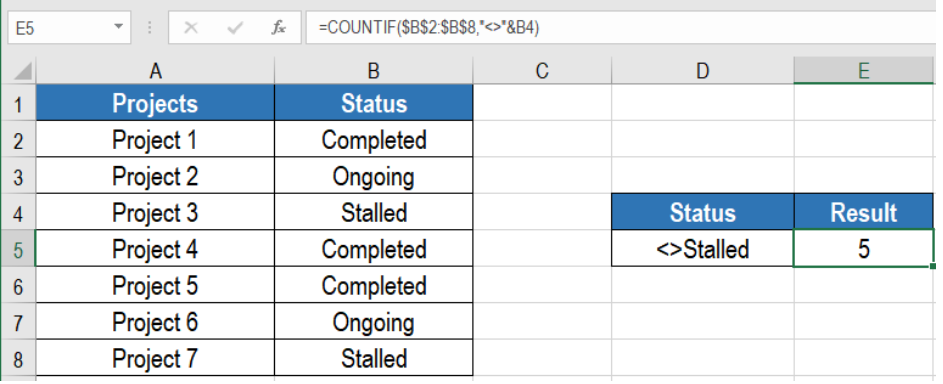Get instant live expert help with Excel or Google Sheets“My Excelchat expert helped me in less than 20 minutes, saving me what would have been 5 hours of work!”

#### Post your problem and you’ll get Expert help in seconds.

Your message must be at least 40 characters
Our professional Expert are available now. Your privacy is guaranteed.

# Count cells not equal to

Many a times we need to count cells based on a condition. However, sometimes the data may require us to count cells that are not equal to a specific value. Excel has a simple solution to count cells not equal to a certain value. We can use the The COUNTIF function can solve this problem very easily. In this article, we will learn how to count cells not equal to another value in Excel.Figure 1. Example of How to Count Cells not Equal to in Excel

## Generic Formula

`=COUNTIF(range, <>value)`

## How this Formula Works

This formula is based on the COUNTIF function. We need to provide the range where we want to count the cells. The next argument is the value that we want to ignore during the count. COUNTIF will count all other values except this one.

First, COUNTIF counts the cells in the range that satisfies the condition we provide. We use the not equal to operator (<>) to count the cells in the range that does not equal to this value. This returns the count of cells other than this value.

## Setting up Data

The following example uses a project information data set. Column A and B has the names and status of the projects. The statuses are “completed”, “ongoing” and “stalled”.Figure 2. The Sample Data Set

To count the cells except the completed ones, we need to:

• Go to cell E4.
• Assign the formula `=COUNTIF(\$B\$2:\$B\$8,”<>Completed”) `to E4.
• Press Enter.Figure 3. Applying the Formula

This will show the count of the projects except the completed ones. We can change this formula to find out the projects except the ongoing and stalled ones as well.

## Notes

1. COUNTIF is not case-sensitive. We can search for any combination of uppercase and lowercase letters.
2. We can use a value from a cell as part of the criteria. To do this we need to use the ampersand character (&)  to do this. To solve the previous example for project that are not stalled, we need to assign the formula `=COUNTIF(B2:B8,"<>"&B4)` to E5.Figure 4. Count Cells not Equal to Using Cell References

This will show the number of projects except stalled in E5.

Most of the time, the problem you will need to solve will be more complex than a simple application of a formula or function. If you want to save hours of research and frustration, try our live Excelchat service! Our Excel Experts are available 24/7 to answer any Excel question you may have. We guarantee a connection within 30 seconds and a customized solution within 20 minutes.

### Did this post not answer your question? Get a solution from connecting with the expert.Another blog reader asked this question today on Excelchat:
Solution examplesI need a formula to count the number of rows that contain at least one of two possible phrases, but not give me the total number of instances that both phrases occur overall.
Solved by E. B. in 33 minsi have a table with Dates, First names , Last names and i want to count how many times a name occurs, but if a name occurs more than once on a particular date i need to only count it once. this is on excel and not google sheets
Solved by F. H. in 31 minsNeed a formula that counts (1,2,3,4 ect..in cell M21) a range of cells, N4:N20, which are annual premium values. But I only want to add a sale count if the average monthly premium is above \$30 per sale. So if someone has 4 sales but they don't equal a total of \$120/mth (\$30 x 4 = \$120)... they would only get a count of 3 sales.
Solved by S. E. in 60 minsHello, I am trying to create a total color column? Can anyone help?
Solved by I. A. in 60 minsAny rep that has orders over 500 and occurrences at zero for each month, for a 6 month period. I need a sum
Solved by C. E. in 60 mins## Subscribe to Excelchat.coAnother blog reader asked this question today on Excelchat: